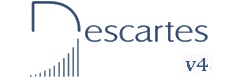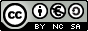Fifth and sixth year of Primary  Education INDEX Introduction. Objectives. 1- Terms of the multiplication. 2- Multiplications. 3- The properties of multiplication. 4- 3x3 square multiplied by a number. 5- 4x4 square multiplied by a number. 6- 3x3 square multiplied by a number. 7- 4x4 square multiplied by a number. 8- 4x4 square multiplied by 4x4 square. 9- 4x4 square formula. 10- Complete a 3x3 square. 11- Complete a 4x4 square. 12- Three-pointed magic star. 1- Divisions. 2- 3x3 square divided by a number. 3- 4x4 square divided by a number. 4- 4x4 square divided by a number. 5- 4x4 square divided by 4x4 square. 1- Powers. 1- Combined operations.
Operations with natural numbers II
INTRODUCTION

In this unit, we revise the basic ideas of natural numbers and we use them to practise the following operations: addition, multiplication, division and powers.

The numbers that appear in each window are generated at random. This allows you to use the window indefinitely and practise a lot of different exercises. Numbers consist of only one or two figures so the calculations can easily be done mentally.

Magic squares with sums and multiplications are used in the exercises, however the main objective of this unit is to learn things about natural numbers.

OBJECTIVES

• To identify values which are natural numbers.
• To understand the concept of multiplication of two natural numbers.
• To make full advantage of the properties of multiplication.
• To understand division is different from multiplication.
• To understand how powers is a repeated multiplication of the same factor.
• To understand and apply properly the hierarchy when combining different operations.
• To train in mental calculation.

 Eduardo Barbero CorralSpanish Ministry of Education. Year 2007